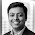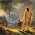## Monday, 16 June 2014

### How HashMap works internally in Java?

One of the most important question of the core java interviewers is How hash map works in java or internal.implementation of hashmap. Most of the candidates rejection chances increases if the candidate do not give the satisfactory explanation . This question shows that candidate has good knowledge of Collection . So this question should be in your to do list before appearing for the interview .
HashMap works on the principle of Hashing .  To understand Hashing , we should understand the three terms first   i.e  Hash Function , Hash Value and Bucket .

### What is Hash Function , Hash Value  and Bucket ?

The hashCode() function  which returns an integer value is the Hash function. The important point to note that ,  this method is present in Object class ( Mother of all class ) .

This is the code for the hash function(also known as hashCode method) in Object Class :

```    public native int hashCode();
```

The most important point to note from the above line :  hashCode method return  int value .
So the Hash value is the int value returned by the hash function .

### What is bucket ?

A bucket is used to store key value pairs . A bucket can have multiple key-value pairs . In hash map, bucket used simple linked list to store objects .

Before start with internal working of HashMap,first you should know this thing,

1. Two unequal object may return same hashcode.
2.  When two objects are equal by equals(), then they must have same hashcode.

There are two main  methods used for storing and retrieving values from HashMap.

1) .put() method to put /store values in HashMap
2) .get() method to retrieve data/values from HashMap

### 1) How put(Key key, Value v) method works internally?

Lets see implementation of put method:

```public V put(K key, V value) {
if (key == null)
Nreturn putForNullKey(value);

int hash = hash(key.hashCode());
int i = indexFor(hash, table.length);
for (Entry<k , V> e = table[i]; e != null; e = e.next) {
Object k;
if (e.hash == hash && ((k = e.key) == key || key.equals(k))) {
V oldValue = e.value;
e.value = value;
e.recordAccess(this);
return oldValue;
}
}
modCount++;
return null;
}
```

Let us understand above code step by step,

1) Key object is checked for null. If key is null then it will be stored at table because hashcode for
null is always 0.
2) Key object’s hashcode() method is called and hash code is calculated. This hashcode is used to find
index of array for storing Entry object. It may happen sometimes that, this hashcode function is
poorly written so JDK designer has put another function called hash() which takes above calculated
hash value as argument.
3) indexFor(hash,table.length)  is used to calculate exact index in table array for storing the Entry
object.
The indexFor will return the index of table array, the index calculation is based on hashcode of key and length of table array. This will do the and operation as ,
` `
``` static int indexFor(int h, int length) {
return h & (length-1);
}```

4) If two key objects have same hashcode(which is known as collision) then it will be stored in form

### 2) How get(Key key) method works internally?

Here are the steps, which happens, when you call get() method with key object to retrieve corresponding value from hash based collection.

Let's see code,

```public V get(Object key) {

if (key == null)
return getForNullKey();

int hash = hash(key.hashCode());
for (Entry<k , V> e = table[indexFor(hash, table.length)]; e != null; e = e.next) {
Object k;
if (e.hash == hash && ((k = e.key) == key || key.equals(k)))
return e.value;
}
return null;
}
```

1) Key object is checked for null. If key is null then value of Object resides at table will be returned.
2) Key object’s hashcode() method is called and hash code is calculated.
3) indexFor(hash,table.length)  is used to calculate exact index in table array using generated hashcode for getting the Entry object.
4) After getting index in table array, it will iterate through linkedlist and check for key equality by calling equals() method and if it returns true then it returns the value of Entry object else returns null.

### What happens if two keys has same hashCode?

If multiple keys has same hashCode, then during put() operation collision had occured, which means multiple Entry object stored in a bucket location. Each Entry keep track of another Entry, forming a linked list data structure. Now , if we need to retrieve value object in this situation, following steps will be followed :

1) Call hashCode() method of key to find bucket location.
2) Traverse  through linked list, comparing keys in each entries using keys.equals() until it return true. So, we use equals() method of key object to find correct entry and then return value from that.

### 6) Hash code for null keys is always zero, and such Entry object is always stored in zero index in Entry[].

Related Posts:--

5) String Interview Questions and Answers
6) Exception Handling Interview questions and answers

1.so why i get such output?

import java.util.HashMap;
public class StudentDemo {

String name;
int id;
public StudentDemo() {

name = null;
id = 0;

}
public StudentDemo( String name , int id){

this.name = name;
this.id = id;
}
public static void main(String arh[]){

StudentDemo s1 = new StudentDemo("shramik",121);
StudentDemo s2 = new StudentDemo("rohit",122);
StudentDemo s3 = new StudentDemo("suresh",123);
StudentDemo s4 = new StudentDemo("karan",124);
StudentDemo s5 = new StudentDemo("pratik",125);
StudentDemo s6 = new StudentDemo("mohan",126);

//System.out.println(s1.hashCode()+" "+s2.hashCode()+" "+s3.hashCode()+" "+s4.hashCode()+" "+s5.hashCode()+" "+s6.hashCode());

HashMap hm = new HashMap();

hm.put(s1,"8982xxxxxx");
hm.put(s2,"8934xxxxxx");
hm.put(s3,"9922xxxxxx");
hm.put(s4,"9835xxxxxx");
hm.put(s5,"8089xxxxxx");
hm.put(s6,"5678xxxxxx");
hm.put(new StudentDemo("abc",127),"8934xxxxxx");

System.out.println(hm.get(s1));
System.out.println(hm.get(s2));
System.out.println(hm.get(s3));
System.out.println(hm.get(s4));
System.out.println(hm.get(s5));
System.out.println(hm.get(s6));
System.out.println(hm.get(new StudentDemo("abc",127)));

}
}
o/p:-
8982xxxxxx
8934xxxxxx
9922xxxxxx
9835xxxxxx
8089xxxxxx
5678xxxxxx
null

i am not override the hashCode() and equals() method also i invoke get(key) method on hashmap object i get respected value but for last key not why?

2.In the above case, While creating new class object you must override the hashCode() and equals() method. While creating new object it will create new hash code that won't match with existing hash code so it will return null

3.One of the most important question of the core java interviewers is How hash map works in java or internal.implementation of hashmap. Most of the candidates rejection chances increases if the candidate do not give the satisfactory explanation . This question shows that candidate has good knowledge of Collection . So this question should be in your to do list before appearing for the interview .
Thanks for helping me to understand basic concepts. As a beginner in Java programming your post help me a lot.Thanks for your informative article.
Thanks a lot! You made a new blog entry to answer my question; I really appreciate your time and effort.
java training in chennai |
java training institutes in chennai